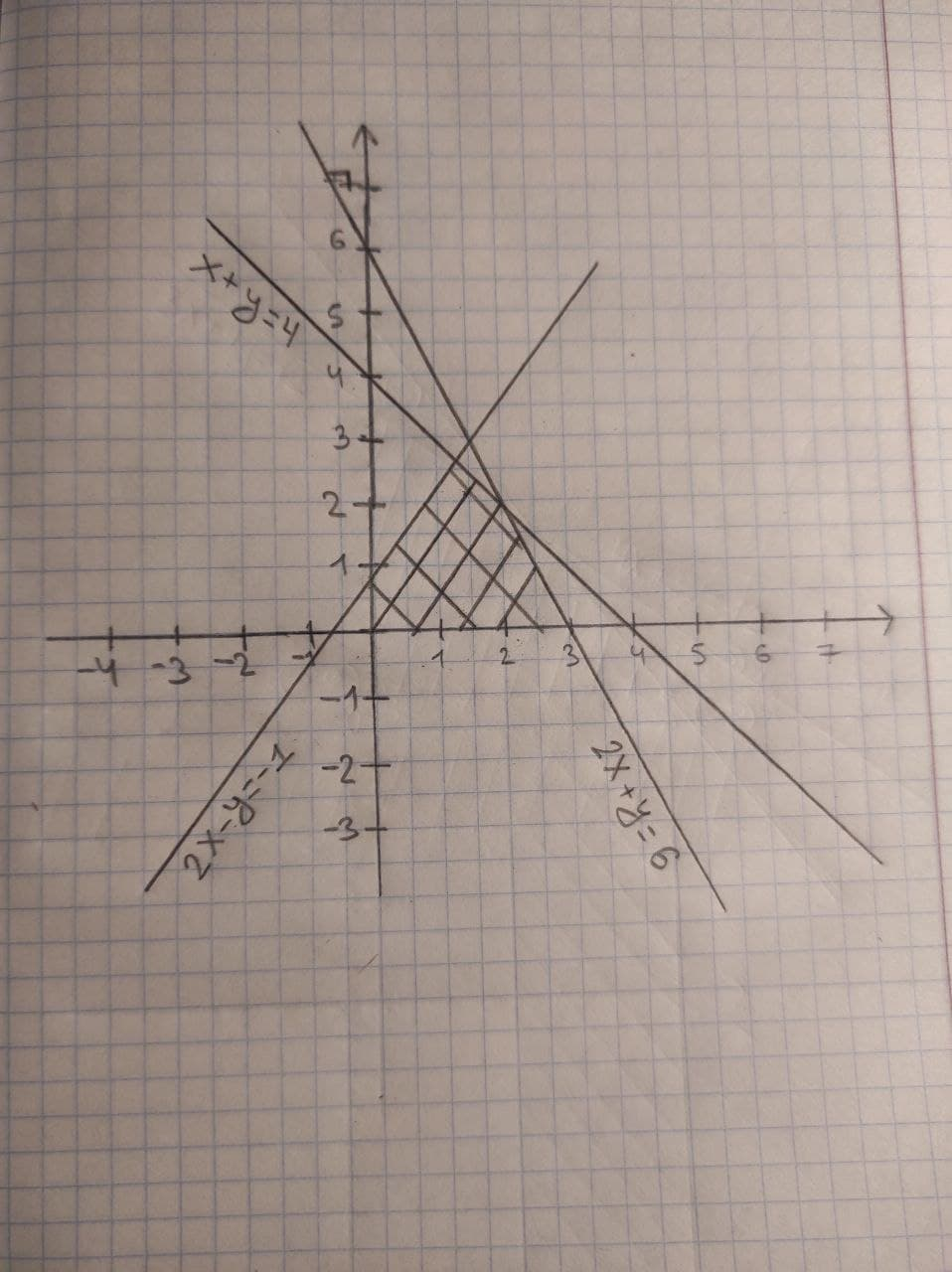# Determine graphically the solution set for the system of inequalities: x+y <= 4 2x+y <= 6 2x−y >= −1 x >= 0, y >= 0FizeauV 2021-02-02 Answered
Determine graphically the solution set for the system of inequalities:
$x+y\le 4$
$2x+y\le 6$
$2x-y\ge -1$
$x\ge 0,y\ge 0$
You can still ask an expert for help

## Want to know more about Inequalities systems and graphs?

• Questions are typically answered in as fast as 30 minutes

Solve your problem for the price of one coffee

• Math expert for every subject
• Pay only if we can solve itsaiyansruleA
Draw the graph of the lines
$x+y=4$
$2x+y=6$
$2x-y=-1$